# What is Algorithm?

Amit Dhanwani on October 18, 2022## What is Algorithm?

“A set of rules to be followed in computations or other issue-solving procedures” or “A process for solving a mathematical problem in a finite number of steps that typically incorporates recursive operations” are two definitions of the term algorithm.

## Introduction

An algorithm is a collection of clear instructions for solving a specific issue in computer programming. It generates the required result from a collection of inputs.

An algorithm can be implemented in more than one programming language since algorithms are typically designed independently of the underlying languages.

Young kids will comprehend what an algorithm is in coding when they are learning how to make their own program.

Alternatively, they will clearly understand algorithm in programming. They will also learn the fundamentals of the Scratch programming language.

An effective approach to describe an algorithm is to state that whoever develops it is setting the rules of the game. As a result, you may explain to your kids that when they create an algorithm, they choose what will happen, which is a very valuable life skill.

By using the example of washing your hands, it may be comprehended. One must follow the directions and carry out the processes one by one in the order specified in order to wash their hands. The finished product is properly cleansed hands. Similar to this, algorithms assist programmers in carrying out tasks to produce desired results.

The developed algorithm in programming is language-independent, meaning that it consists of simple instructions that may be used to build it in any language and still provide the desired results.

## Flow chart

A formal definition of an algorithm is that it is a finite set of instructions that are carried out in a certain order to complete a specified task.

Simple logic to a problem is offered as an informal explanation in the form of a flowchart or pseudocode; it is not the whole program or code.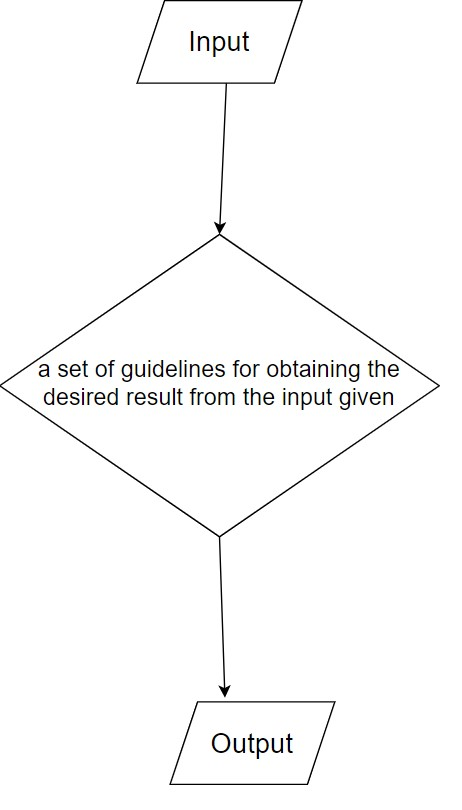Figure 1: Flow chart of the algorithm

Problem Statement: A real-world problem or a real-world instance problem for which you need to create a program or a set of instructions is what is referred to as a problem. An algorithm is a group of rules.

Algorithm: An algorithm is described as a method that will be used to solve a problem step-by-step.

Input: Once an algorithm has been created, the required and preferred inputs are provided to the algorithm.

Processing unit: The processing unit will receive the input and produce the required output.

Output: The output of a program is referred to as its result or outcome.

## What makes a good Algorithm?

1. It’s important to specify input and output precisely.
2. The algorithm’s steps should all be distinct and unambiguous.
3. The most efficient technique to tackle a problem should be an algorithm.
4. Computer code shouldn’t be used in an algorithm. The technique should instead be constructed so that it may be applied to other programming languages.

## Algorithm characteristics

• Input: An algorithm needs certain input values, which need to be clearly described. A value other than 0 may be provided as input to an algorithm.
• Output: An algorithm will provide one or more outputs when it is finished.
• Unambiguity: According to the definition of a good algorithm, its instructions must be unambiguous, which calls for simple and straightforward language. And can only have one meaning.
• Finiteness: A finite algorithm is required. In this context, “finiteness” refers to the property that an algorithm should contain a finite amount of instructions, be countable, and end after a finite period of time.
• Effectiveness: An algorithm’s instructions should be sufficient since they have an impact on the entire process.
• Feasible: The method must be straightforward, general, and workable so that it may be implemented using the resources at hand. It can’t have any cutting-edge technology or anything.
• Language Independence: An algorithm must be language-independent, which implies that its instructions must work the same no matter what language is used to implement them.

## Simple example to demonstrate Algorithm

Create a multiplier algorithm that displays the result of multiplying two integers.

Step 1 − Start

Step 2 − Initialize three integers num1, num2 & result

Step 3 − Assign values to num1 & num2

Step 4 − Multiply values of num1 & num2

Step 5 − store the answer of step 4 in the result integer

Step 6 − Print the result

Step 7 – Stop

The use of algorithms helps programmers how to write code.

This algorithm can also be expressed as follows:

Step 1 − Start

Step 2 − Initialize three integers num1, num2 & result

Step 3 − Assign values to num1 & num2

Step 4 − Start mul

Step 5 − get values of num1 & num2

Step 6 − result ← num1* num2

Step 7 − display result

Step 8 – Stop

## Considerations while developing the Algorithm

The following considerations must be made when developing the algorithm:

• Accuracy: An algorithm is said to be accurate when the provided inputs result in the expected output, which shows that the method was properly developed. The analysis of an algorithm has been correctly finished.
• Reusability: This refers to the idea that the algorithm should be created in a simple, organized manner so that when it is redefined, it does not undergo substantial changes.
• Modularity: If you are given a problem and break it down into small-small modules or small-small stages, which is a basic description of an algorithm, this characteristic was perfectly designed for the algorithm.
• Adaptability: If another algorithm designer or programmer wants to utilize your algorithm, it should be adaptable.
• Functionality: It considers several logical procedures in order to resolve a practical issue.
• Robustness: The term robustness refers to an algorithm’s capacity to precisely characterize your issue.
• Simplicity: An algorithm is simple to understand if it is simple.
• User-friendly: The designer won’t be able to communicate the method to the coder if it is difficult to understand.

## Important types of Algorithms

Different sorts of algorithms are employed to address computing issues in a variety of ways. The ones that are most frequently used are:

• Brute Force Algorithm: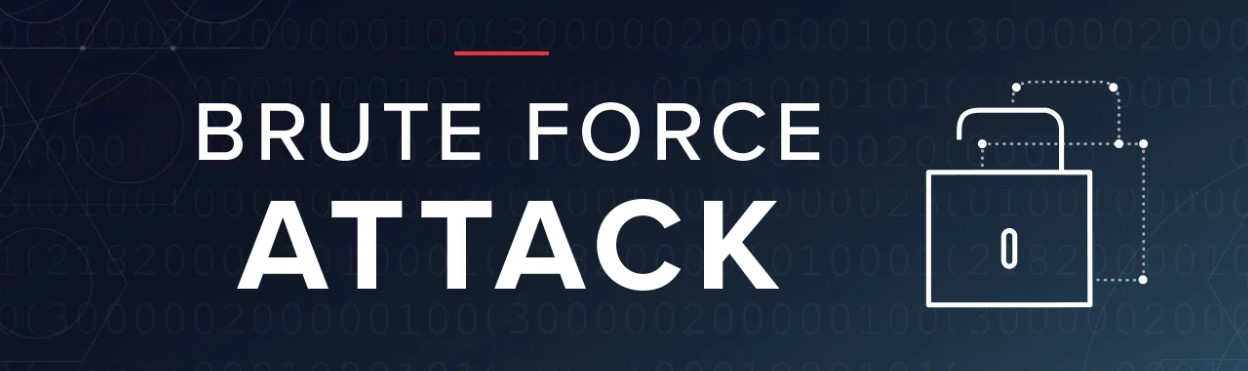It is the easiest solution to the problem. When we see a problem, the first method that comes to mind is a brute force algorithm.

The general logic structure is used in this algorithm’s development. It is also known as an extensive search algorithm since it explores every possibility before delivering the needed output.

These algorithms come in two varieties:

1. Optimizing: Finding every possible solution to a problem, then choosing the best one; if the best answer is found, the process will end.
2. Sacrificing: Once the best solution is identified, it will come to an end.
• Divide and Conquer Algorithm:As the name suggests,  This method divides a problem into smaller problems, solves each smaller problem in succession, then combines the results to provide the overall solution. The procedure includes the following three steps:

1. Divide
2. Solve
3. Combine
• Greedy Algorithm: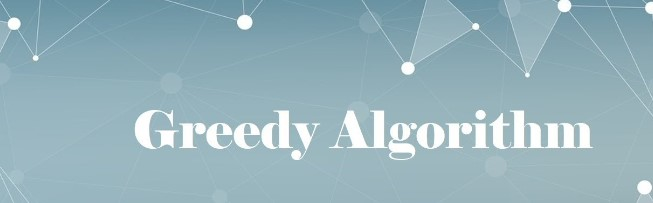This kind of algorithm builds the solution one by one. It takes less time to execute and is easy to set up.

Greedy algorithms use a method of problem-solving that proceeds gradually and chooses the action to take that provides the most profit immediately.

Although it attempts to discover the most locally optimum solution, it frequently falls short since it does not examine all of the data.

• Dynamic Programming: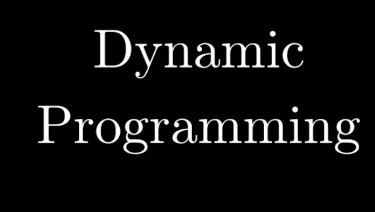The idea behind this approach is to avoid repeating calculations for the same part of the problem by applying the previously found answer. It separates the issue into more manageable overlapping subproblems and resolves each one.

• Recursive Algorithm:It is an algorithmic process that iteratively discards solutions that do not adhere to the problem’s requirements. In this situation, a problem is divided into multiple smaller components and repeatedly called by the same function.

• Backtracking Algorithm:The backtracking algorithm basically constructs the solution by looking through all potential solutions. This program breaks down any problem into its sub components and resolves them.

We go back to the failure point whenever a solution fails, build on the next one, and repeat this process until we either discover the answer or have considered every option.

• Searching Algorithm:The algorithms used for searching individual components or groups of elements from a certain data structure are called searching algorithms. Depending on how they go about tasks or whatever data structure the element has to be in, they can be of several types.

• Sorting Algorithm:Sorting is the process of organizing a collection of data in a certain way in accordance with the requirements. Sorting algorithms are the ones that help in carrying out this task. Data groupings are often sorted using sorting algorithms in an increasing or decreasing order.

• Hashing Algorithm: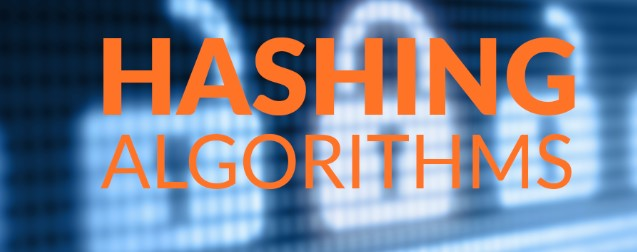Similar to how a search algorithm operates, hashing methods do the same. Nevertheless, they have an index with a key ID. In hashing, a key is given to a particular piece of data.

• Randomized Algorithm: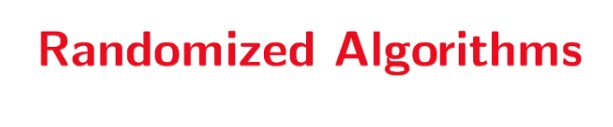We use a random integer in the randomized process so that it provides a quick advantage in finding the solution to the problem. The predicted result is determined in part by the random number.

## Benefits of Algorithms

• It is simple to comprehend.
• A solution to a problem is represented step-by-step in an algorithm.
• Since the problem is divided into smaller components or stages when using an algorithm, it is simpler for the programmer to convert the algorithm into a working program.

## The drawbacks of Algorithms

• It takes a lot of time to write an algorithm, hence it is time-consuming.
• It can be quite challenging to comprehend complicated reasoning using algorithms.
• It is challenging to demonstrate branching and looping statements in algorithms.

## Some more examples of Algorithms

• Find the largest number among three numbers:

Step 1: Start

Step 2: Declare & Initialize variables num1, num2, and num3.

Step 3: Read variables num1, num2, and num3.

Step 4: If num1 > num2

If num1 > num3

Display num1 is the largest number.

Else

Display num3 is the largest number.

Else

If num2 > num3

Display num2 is the largest number.

Else

Display num3 is the greatest number.

Step 5: Stop

• Find the factorial of a number:

Step 1: Start

Step 2: Declare & Initialize variables n, factorial, and i.

Step 3: Initialize variables

factorial ← 1

i ← 1

Step 4: Read the value of n

Step 5: Repeat the steps until i = n

5.1: factorial ← factorial*i

5.2: i ← i+1

Step 6: Display factorial

Step 7: Stop

• Check whether a number is prime or not:

Step 1: Start

Step 2: Declare variables n, i, flag.

Step 3: Initialize variables

flag ← 1

i ← 2

Step 4: Read n from the user.

Step 5: Repeat the steps until i=(n/2)

5.1 If remainder of n÷i equals 0

flag ← 0

Go to step 6

5.2 i ← i+1

Step 6: If flag = 0

Display n is not prime

else

Display n is prime

Step 7: Stop

• Find the Fibonacci series till the term less than 500:

Step 1: Start

Step 2: Declare variables first_term, second_term, and temp.

Step 3: Initialize variables first_term ← 0 second_term ← 1

Step 4: Display first_term and second_term

Step 5: Repeat the steps until second_term ≤ 500

5.1: temp ← second_term

5.2: second_term ← second_term + first_term

5.3: first_term ← temp

5.4: Display second_term

Step 6: Stop

• Find Roots of a Quadratic Equation ax2 + bx + c = 0:

Step 1: Start

Step 2: Declare variables a, b, c, D, x1, x2, rp, and ip;

Step 3: Calculate discriminant

D ← b2-4ac

Step 4: If D ≥ 0

r1 ← (-b+√D)/2a

r2 ← (-b-√D)/2a

Display r1 and r2 as roots.

Else

Calculate the real part and imaginary part

rp ← -b/2a

ip ← √(-D)/2a

Display rp+j(ip) and rp-j(ip) as roots

Step 5: Stop

• N Queens problem using backtracking algorithm:

The plan is to arrange the queens one at a time in the various columns, beginning with the leftmost column.

When we place a queen in a column, we look to see if any other queens have previously been placed.

If a row for which there is no collision is found in the current column, we record this row and column as being a component of the solution.

We go back and return false if we cannot locate such a dispute because of conflicts.

Step 1: Start in the leftmost column

Step 2: If all queens are placed

return true

Step 3: Test every row in the present column.

For each attempted row, carry out the following..

Step 3.1: If this row can accommodate the queen securely,

thereafter, add this [row, column] to the

solution and repeatedly determining if placing

queen here leads to a solution.

Step 3.2: If placing the queen in [row, column] leads to

a solution then returns true.

Step 3.3: If placing queen doesn’t lead to a solution then

unmark this [row, column] (Backtrack) and go to

step 3.1 to try other rows.

Step 4: If after attempting each row, nothing was successful,

return false to start the backtracking process.

## Conclusion

Understanding algorithms is crucial because it enables students to break down problems and construct solutions as distinct processes.

In the end, algorithms serve as the foundation for all computer operations. Since they can efficiently complete repetitive activities and automate various jobs, algorithms are essential for all computerized devices since they can streamline the entire process. They can reduce costs and save time.

Python for kids can open up a lot of career options for kids in the future. Learning Python now will help them develop skills that are essential for life. With the rise of artificial intelligence and automation, computational thinking and coding literacy has become a required skill of the twenty-first century across all industries.

With many advanced fields using Python, a lot of career opportunities have opened up now and will continue to do so in the future. It is used in many fields and is expected to continue to do so. Python knowledge can open up a lot of career options for your kid in the future.

Coding for kids encourages children’s creativity and development by allowing them to experiment and gain confidence in their ability to innovate. Try a free class today!